Click to Chat

1800-1023-196

+91-120-4616500

CART 0

• 0

MY CART (5)

Use Coupon: CART20 and get 20% off on all online Study Material

ITEM
DETAILS
MRP
DISCOUNT
FINAL PRICE
Total Price: Rs.

There are no items in this cart.
Continue Shopping```Resolution of a Quadratic Function into Linear Factors0Let f(x, y) = ax2 + 2hxy + by2 + 2gx + 2fy + c (a = 0, b = 0)

writing this in descending powers of y and equating to zero, we have by2 + 2(hx + f)y + (ax2 + 2gx + c) = 0

this is a quadratic equation in y.

Solving this for y, we get

y =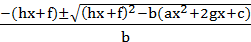Þ     (hx + by + f) = +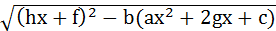Now, in order that f(x, y) may be the product of two linear factors of the form px + qy + r; the quantity under radical must be perfect square, which is quadratic in x and for the desired result, its discriminant must be zero, i.e.,

(hf – bg)2 = (h2 – ab)(f2 – bc)

h2f2 + b2g2 – 2hfg = h2f2 – h2bc – abf2 + ab2c

abc + 2fgh – af2 – bg2 – ch2 = 0

which is the condition required.

Illustration:

Prove that f(x, y) = 2x2 – 3xy – 2y2 + 5x + 2 can be resolve into two         linear factors.

Solution:

Here, a = 2, b = –2, c = 2, f = 0, g =and h =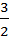.

So, a b c + 2 f g h – af2 – bg2 – ch2 = 8 + 0 + 0 +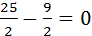Hence given quadratic function can be resolve into two linear factors.

Solved Examples

Example 1:

Let a, b be the roots of the equation ax2 + bx + c = 0, given that
b2 – 4ac > 0, show that

(i)     both a and b are positive when ‘a’ and ‘c’ are of the same sign and ‘b; is of opposite sign

(ii)    both a and b are negative when a, b, c are all of the same sign

(iii)    a and b are both real and of opposite sign when ‘a’ and ‘c’ are of opposite sign irrespective of ‘b’.

(iv)   a and b are equal in magnitude but opposite in sign when b = 0

Solution:

(i)     Since ‘a’ and ‘c’ are of same sign, so a.b must be positive. This is possible only when both have same sign. Since a + b = –b/a = (positive)(because a & b are of opp. Sign). So a and b must be both positive.

(ii)    Here again a.b = c/a = +ve and a + b = –b/a hence either both roots are positive or both are –ve. As a + b = –b/a = positive. This is only possible when a & b both are negative.

(iii)    When ‘a’ and ‘c’ are of opposite sign ac is negative. Further b2 is always positive. Hence b2–4ac remains positive and value of positive square root of b2 – 4ac will be more than b. So addition and subtraction with negative ‘b’ will give different sign to the roots.

(iv)   When b = 0, –b/a = 0. We therefore have a + b = 0, i.e. a = –b, thus the roots are equal in magnitude but opposite in sign.

Example 2:

If P(x) = px2 + qx + r, Q(x) = –px2 + qx + r where pr ¹ 0, then show that P(x) Q(x) = 0 has at least two real and distinct roots.

Solution:

For real roots, D > 0 i.e. b2 – 4ac > 0

In this case D = q2 – 4pr

so, in case of pr < 0, D will always be positive (because b2 > 0)

Since the coefficient of x2 in two equations are equal and of opposite sign, so, discriminant at least one of the given two quadratic equations must be greater than zero. So P(x) Q(x) = 0 must have at least two distinct roots.

Example 3:

Solve |x2 + 4x + 3| + 2x + 5 = 0.

Solution:

|x2 + 4x + 3| + 2x + 5 = 0

Þ |x2 + 3x + x + 3| + 2x + 5 = 0

Þ |x(x + 3) + (x + 3)| + 2x + 5 = 0

Þ |(x + 1)(x + 3)|+ 2x + 5 = 0

Case I:

–3 < x < –1

then, given equation will becomes

–(x + 1)(x + 3) + 2x + 5 = 0

–x2 + 4x – 3 + 2x + 5 = 0

x2 + 2x – 2 = 0

x =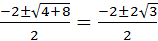= –1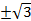since x Î [–3, –1]

so x = –1 –Case II:

x Î x Î (–¥, –3 and x Î –1, ¥)

then given equation will become

x2 + 4x + 3 + 2x + 5 = 0

or     x2 + 6x + 8 = 0

x =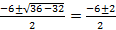= –3 + 1 = –4, –2

Since –2 Î [–µ, –3]

So only solutions in this case is –4

So all possible solutions are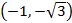& –4

Example 4:

Find the solution set for the equation

2 cos2sin2 x = x2 + x–2, x > 0

Solution:

Since x2 > 0

So     x2 + x–2 = x2 +> 2

Þ 2 cos2sin2x > 2

Þ cos2sin2x > 1                                               …………… (i)

but value of cos2and sin2x always lie in [0, 1] so

cos2. sin2 x < 1                                                …………… (ii)

The inequalities (i) and (ii) lead to equality

cos2. sin2 x = 1

Þ x2 += 1

Þ x2 – 2x2 + 1 = 0

Þ x2 = 1

or     x = + 1

but for x = 1 or –1 (taken at random)

cos2. sin2x < 1

Hence LHS and RHS of this equation can never be equal. Hence there is no solution.

Example 5:

Show that

f(x) =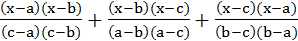– 1 = 0 is an identity where a, b, c are real and distinct numbers.

Solution:

f(x) is quadratic in nature, so f(x) = 0 must have two solution.

Since f(a) = f(b) = f(c) = 0

i.e., a, b, c all are solutions of equation (x) = 0

We know that when a polynomial equation of degree n has (n + 1) roots then it becomes an identity. Here a, b, c are distinct and f(x) = 0, a quadratic equation has 3 roots therefore f(x) = 0 is an identity which gives constant value (i.e. 0) for any value of x.

Note:

Whenever a polynomial equation of degree n has more than n roots then it turns out to be an identity.

Example 6:

If a, b, are the roots of x2 + px + 1 = 0 and c, d are the root of x2 + qx + 1 = 0 show that q2 – p2 = (a – c)(b – c)(a + d)(b + d)

Solution:

Since a, b, are the roots of x2 + px + 1

So     a + b = –p; ab = 1

Similarly c + d = –q; cd = 1                                         …… (i)

\ R.H.S. = (a – c)(b – c)(a + d)(b + d)

= (ab – ac – bc + c2)(ab + ad + bd + d2)

= {ab – c (a + b) + c2} {ab + d (a + b) + d2}

= (1 + pc + c2)(1 – pd + d2)(from (i))

= (cd + pc + c2)(cd – qd + d2)(ab = cd = 1)

= {(d + c + p} d {(c + d) – q}

= cd (–q + p)(–q – p

= –(p – q)(p + q)

= q2 – p2

Example 7:

If one root of the equation ax2 + bx + c = 0 be the square of the other, then show that a (c – b)3 = c(a – b)3

Solution:

Let a and a2 be the roots of the equation ax2 + bx + c

\a + a2 = –b/a and a3 = c/a

Since a3 – b3 = (a – b)(a2 + ab + b2)

So     a3 – 1 = (a – 1)(a2 + a + 1)

c/a – 1 = (a – 1)((–b/a)+1)

Þa = 1 +\a3 =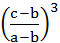in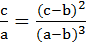(a3 = c/a)

or     a(c – b)3 = c(a – b)3

Example 8:

If a, b are the roots of the equation ax2 + bx + c = 0. Find the equation whose roots are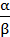and.

Solution:

We have,           a + b =ab =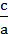Now sum of the roots =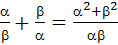===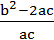and product of the roots =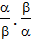= 1

so the required equation is

x2 – (sum of the roots) x + (product of the roots) = 0

Þ     x2 –x + 1 = 0

Þ     ac x2 – (b2 – 2ac) x + ac = 0

Example 9:

Prove that the minimum value of, where x > –c.

Solution:

Let    y =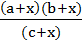=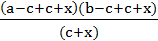=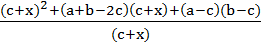= (c + x) +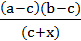+ (a + b – 2c)

=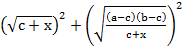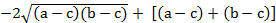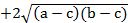=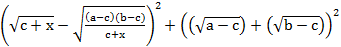so ymin =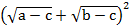Example 10:

If x be real, prove that the expression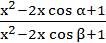lies between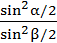and.

Solution:

Let y =Rearranging

(1 – y) x2 – 2(cosa – y cosb) x + (1 – y) = 0

Since x is real so D > 0

\ 4(cos a – y cos b)2 –4(1 – y)2 > 0

Þ     (cosa – y cosb)2 –(1 – y)2 > 0

Þ     (cosa – y cosb + 1 – y)(cosa – y cosb – 1 + y) > 0

Þ     [(1 + cosb)y –(1 + cosa)][(1 – cosb)y–(1 – cosa)] > 0

\ y lies between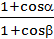andi.e. betweenand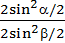i.e. between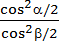and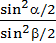.

Example 11:

Find all integral values of x for which (5x – 1) < (x + 1)2 < (7x – 3)

Solution:

We have

(x + 1)2 > 5x – 1

or     x2 – 3x + 2 > 0

i.e.    (x – 1) (x – 2) > 0

\ x > 2

Again (x + 1)2 < 7x – 3

i.e.    (x –1) (x – 4) < 0

\ x < 4

Hence the only integral solution is x = 3.

Example 12:

a1, a2 are roots of the quadratic equation ax2 + bx + c = 0 and b1, b2 are that of px2 + qx + r = 0. Further, the system of linear equations       a1x + b1y = 0, a2x + b1y = 0 have a non-trivial solution. Then prove   that.

Solution:

We have a1, a2 =, a1.a2 =b1 + b2 =, b1.b2 =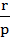Further, the given system has already one trivial solution (0, 0) and according to question, it has one more solution which is non-trival. But system of distinct linear equation can have at the most one solution. Therefore, the given equation must represent the line. For this, the coefficient must be in the same ratio, i.e.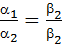Þ(Using properties of ratios)

Þ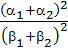ÞTo read more, Buy study materials of Quadratic Equation comprising study notes, revision notes, video lectures, previous year solved questions etc. Also browse for more study materials on Mathematics here.
```### Course Features

• 731 Video Lectures
• Revision Notes
• Previous Year Papers
• Mind Map
• Study Planner
• NCERT Solutions
• Discussion Forum
• Test paper with Video Solution